## Section14.3Normal Modes of a String

Normal modes of strings help us understand the sound produced by plucking guitar strings and other string instruments. Consider a string tied at both ends. First, we divide up the mass of the string in $N$ parts and place them as beads on equal intervals as shown in Figure 14.3.1.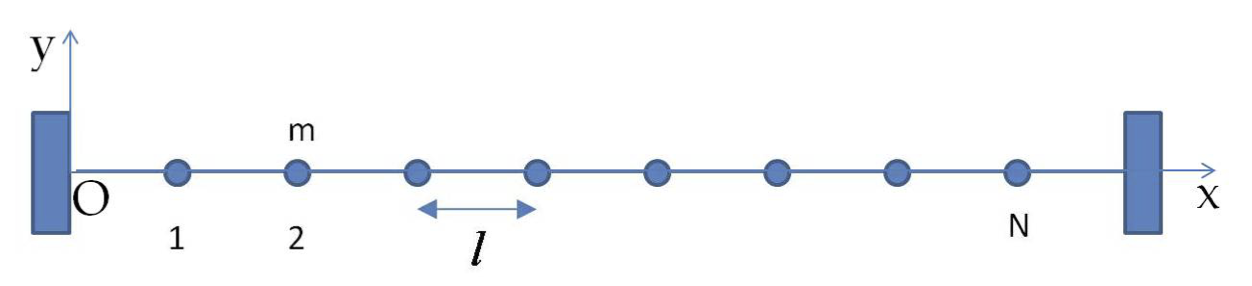Figure 14.3.1. $N$ beads on a taut string act as $N$ coupled oscillators.

This converts the problem of vibration of a string to the vibrations of $N$ beads, each of mass $m\text{,}$ on a massless string which are coupled by the tension force in the string. For the sake of ease of visualization of the modes we consider the modes of the transverse motion of the string.

Before we discuss the motion of this general model of a string, let us look a simpler case of two beads on a string.

### Subsection14.3.1(Calculus) Motion of Two Beads on a Taut String

Now, consider two beads on a string. For simplicity, we will take their masses to be same, $m\text{,}$ and place them symmetrically. Let $l$ be the distance between the beads and on either side of the two beads. That is length of the string, $L=3l$ here, as shown in Figure 14.3.2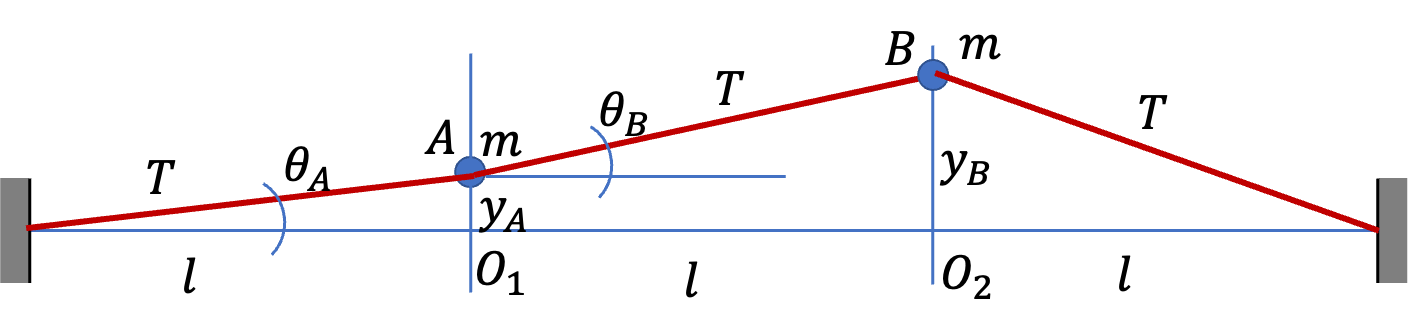Figure 14.3.2. Two beads A and B placed symmetrically on a taut string with tension $T$ shown at an arbitrary instant with vertical displacments $y_A$ and $y_B\text{.}$ We study only the vertical motion here.

Rather than study the general motion, we will find only the normal modes of the system in the small displacment approximation, i.e., Assume small displacements, i.e., when $|y_A| \lt\lt l$ and $|y_B|\lt\lt l\text{,}$ where $y_A$ and $y_B$ are displacements of the beads.

You should show that the $y$ component of net force on the two beads are

\begin{align*} \amp F_{Ay} = \frac{T}{l}\left( -2 y_A + y_B\right)\\ \amp F_{By} = \frac{T}{l}\left( -2 y_B + y_A\right) \end{align*}

This gives following for their accelerations.

\begin{align} \amp \frac{d^2y_A}{dt^2} = \frac{2T}{ml}\left( - y_A + 0.5 y_B\right)\label{eq-of-motion-two-beads-on-string-1}\tag{14.3.1}\\ \amp \frac{d^2y_B}{dt^2} = \frac{2T}{ml}\left( - y_B + 0.5 y_A\right)\label{eq-of-motion-two-beads-on-string-2}\tag{14.3.2} \end{align}

In normal modes, both beads have the frequency of the normal mode. Each bead acts like a simple harmonic oscillator at the frequency of the mode. Let $\omega$ be the frequency of the mode, which we don't know yet - it will be replaced by $\omega_1$ and $\omega_2$ for the two modes.

How do we find $\omega_1$ and $\omega_2\text{?}$ We will just plug in expected behavior of beads in the equations, Eqs. (14.3.1) and (14.3.2). That is, we set

\begin{align*} \amp y_A = A_1 \cos(\omega t) \\ \amp y_B = A_2 \cos(\omega t) \end{align*}

This will be their motions if we start the beads in one of the modes, letting then go at rest - once again, of course $\omega$ will be either $\omega_1$ or $\omega_2\text{,}$ the angular frequencies of the modes. After plugging these in Eqs. (14.3.1) and (14.3.2), we will find these allowed values of $\omega$ and the ratio of $A_2$ to $A_1\text{.}$

To simplify writing, I will introduce a scale for angular frequency by

\begin{equation*} \omega_0 = \sqrt{ \frac{2T}{ml} }, \end{equation*}

and

\begin{equation*} \tilde\omega = \frac{\omega}{\omega_0}. \end{equation*}

The result is

\begin{align} \amp (1-\tilde\omega^2) A_1 = 0.5 A_2 \label{eq-normal-modes-two-beads-on-string-1}\tag{14.3.3}\\ \amp (1-\tilde\omega^2) A_2 = 0.5 A_1\label{eq-normal-modes-two-beads-on-string-2}\tag{14.3.4} \end{align}

Taking the ratio that cancel out $A_1$ and $A_2\text{,}$ we get

\begin{equation*} (1-\tilde\omega^2)^2 = \left( \frac{1}{2}\right)^2. \end{equation*}

This gives two values for $\tilde\omega^2\text{,}$ namely,

\begin{equation*} \tilde\omega_1^2 = \frac{1}{2},\ \ \tilde\omega_2^2 = \frac{3}{2}. \end{equation*}

Therefore, angular frequencies of the normal modes are

\begin{equation} \omega_1 = \sqrt{ \frac{T}{ml} },\ \ \omega_2 = \sqrt{ \frac{3T}{ml} }.\tag{14.3.5} \end{equation}

Putting $\tilde\omega_1$ in Eq. (14.3.3), we see that the ratio of $A_2$ to $A_1$ is

\begin{equation} \left[ \frac{A_2}{A_1}\right]_\text{mode 1} = 1.\tag{14.3.6} \end{equation}

Putting $\tilde\omega_2$ in Eq. (14.3.3), we see that the ratio of $A_2$ to $A_1$ is

\begin{equation} \left[ \frac{A_2}{A_1}\right]_\text{mode 2} = -1.\tag{14.3.7} \end{equation}

Thus, we have our modes now. the frequencies and displacements of the two modes when started from rest are

\begin{align*} \amp \omega_1= \sqrt{ \frac{3T}{mL} }, \ \ \ y_A = y_B = A \cos(\omega_1 t)\\ \amp \omega_2= 3\sqrt{ \frac{T}{mL} },\ \ \ y_A = -y_B = A \cos(\omega_2 t) \end{align*}

We can sketch these normal modes to get the shape of string as the two beads move in one or the other mode. In mode 1, the beads move with the same displacement since $y_A=y_B\text{.}$ Therefore, the string will look like a trapezoid at an arbitrary insant. In mode 2, the beads move with opposite displacments since $y_A=-y_B\text{.}$ Therefore, the string will have a zig-zag shap.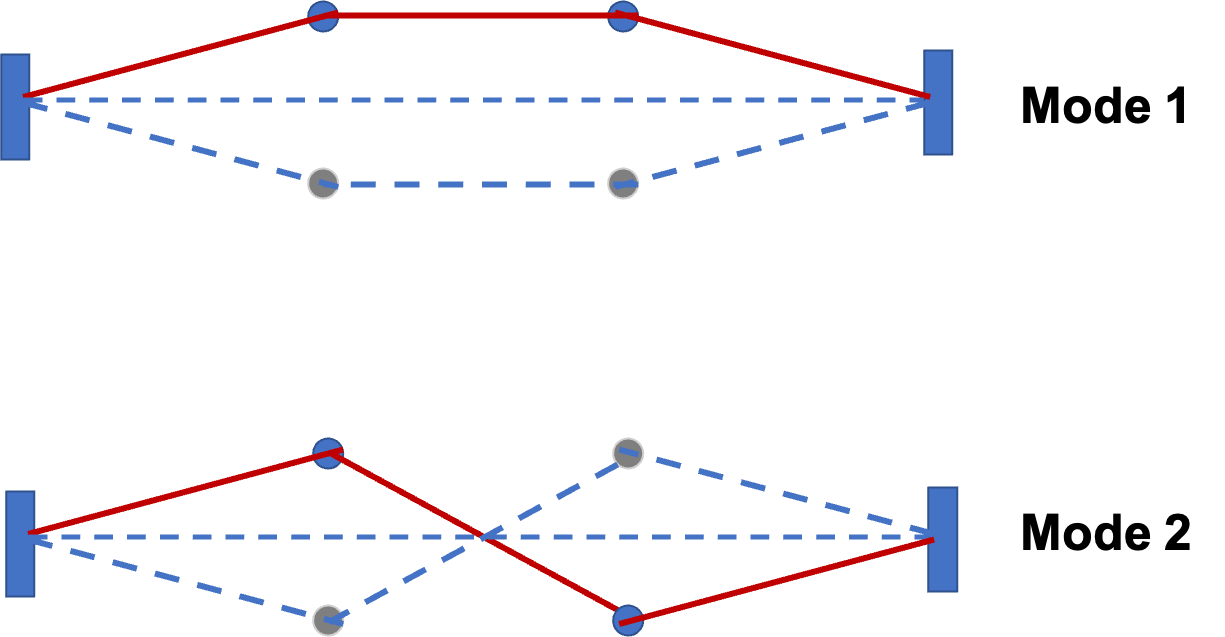Figure 14.3.3. Two normal modes of two beads on a taut string. In mode 1, the two beads move with the same displacment, and in mode 2, they move in the opposite direction with opposite displacements.

### Subsection14.3.2Normal Modes of a String

It is interesting to ask: what will be the normal modes if we have 3, 4, 5, ..., beads on the string. As an illustration, modes of the $N=2,3,4$ on a taut string system are shown in Figure 14.3.4.

You will notice that number of normal modes equals number of these beads and in each mode the ratio of the amplitudes of displacements of different beads must have a particular relation. For instance, in the 3-bead system, we have the following relations between the displacments of the beads.

\begin{align*} \amp \text{Mode 1: } A_1 = B_1 = C_1. \\ \amp \text{Mode 2: } A_2 = -C_2,\ B_2=0. \\ \amp \text{Mode 3: } A_3 = -\frac{1}{\sqrt{2}}B_3 = C_3. \end{align*}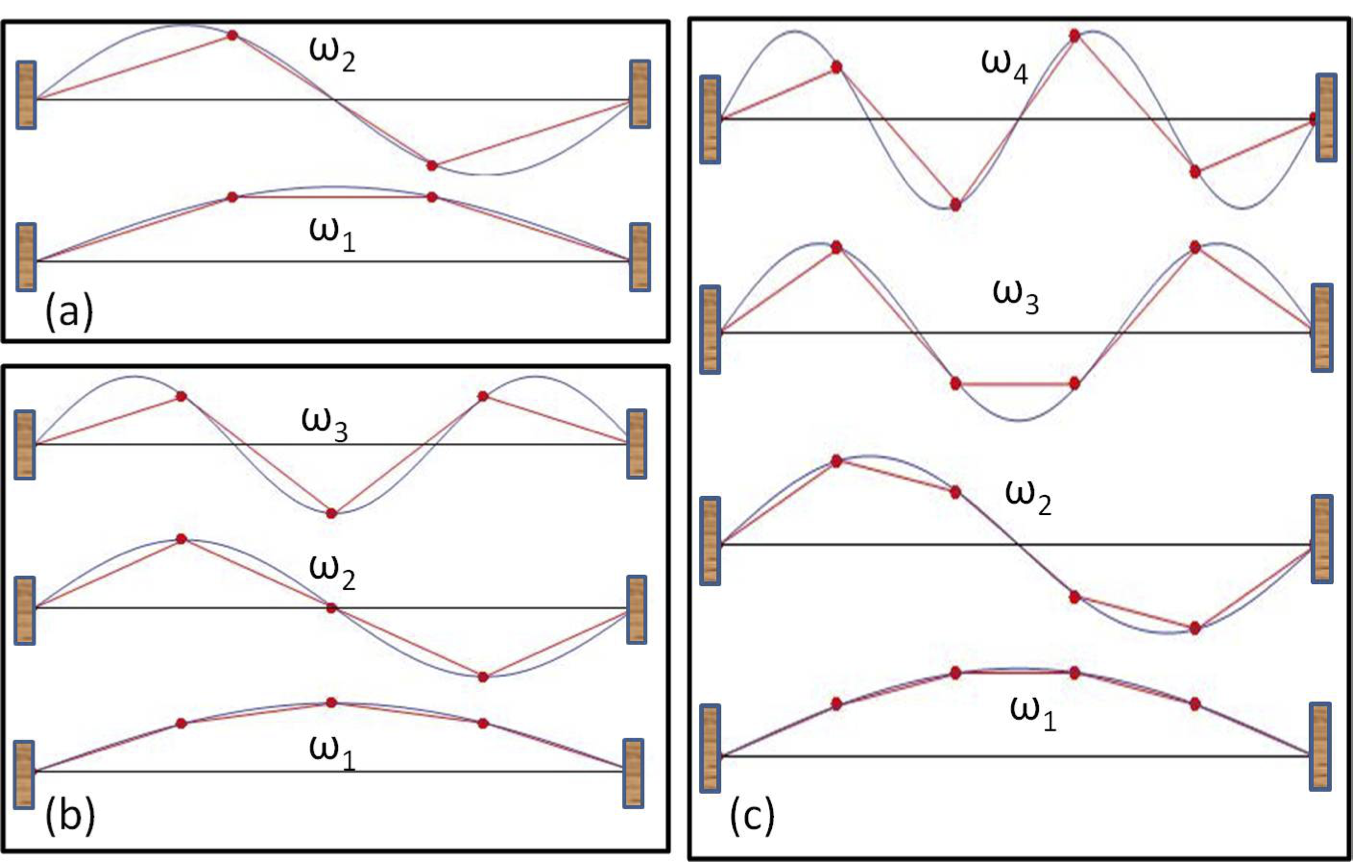Figure 14.3.4. Transverse modes of (a) $N=2\text{,}$ (b) $N=3\text{,}$ and (c) $N=3\text{.}$ In each case $\omega_1$ is the lowest frequency mode. The points at the nodes, the place where the string crosses the zero displacement, do not oscillate; other points of the string oscillate up and down with the frequency of the corresponding normal mode.

As you place more and more beads on the string, you can imagine, at some point, we can just say that beads are just continuously placed on the string. This is $N\rightarrow\infty$ limit. In that case, the shapes of normal modes are just piecess of sine function of increasing frequency.

You will find that the lowest frequency mode has the shape of half cycle of a sine function, the next has the shape of one cycle of a sine function, the next one has $\frac{3}{2}$ cycles, and so on. First three of these modes are shown in Figure 14.3.5. If we imagine these shapes continue in both directions for ever, we will find that they repeat in space. The smallest repeat distance is called wavelength of the mode. We usually denote wavelength by the Greek letter $\lambda$ (lambda).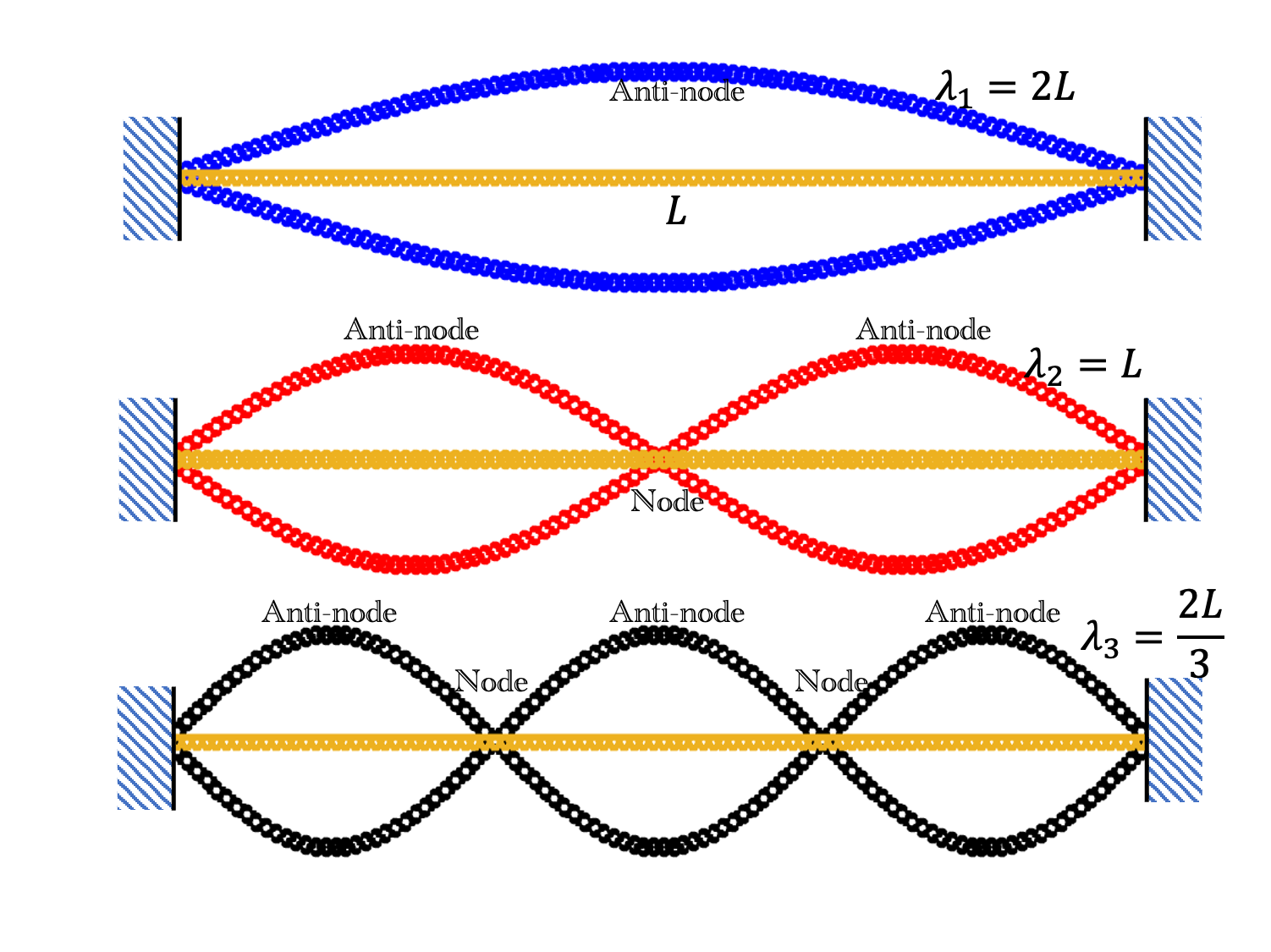Figure 14.3.5. Normal modes of a string tied at both ends. The figure shows modes of lowest three frequencies. These modes are the first three harmonics or the fundamental, the first overtone, and the second overtones. The wavelengths are $\lambda_1 = 2 L\text{,}$ $\lambda_2 = L\text{,}$ and $\lambda_3 = \frac{2L}{3}\text{.}$ The locations of maximum displacment are called anti-nodes and the locations of zero displacement are called nodes. The $\lambda_1$ mode is the fundamental mode and $\lambda_2$ and $\lambda_3$ are overtones.

We can describe the shape of all normal modes by a common formula for shape functions $C_n(x)$ with $n=1, 2, 3, ...$ for the modes. I will state the results here without full calculations since they are involved and you can find them in a book on more advanced books on vibrations and waves.

\begin{equation} C_n(x) = A_n \sin\left( \dfrac{n\pi x}{L} \right).\label{eq-largeN-mode-functions-N-beads-on-string}\tag{14.3.8} \end{equation}

Each part of the string will be vibrating in a simple harmonic motion with the frequency of that mode. Therefore, at any instant $t\text{,}$ the string in the $n$th mode will have the shape given by

\begin{equation} y_n(x,t) = C_n(x) \cos(\omega_n t) = A_n \sin\left( \dfrac{n\pi x}{L} \right) \cos(\omega_n t),\tag{14.3.9} \end{equation}

where angular frequencies are

\begin{equation} \omega_n = n \omega_1, \ \text{ with }\ \omega_1 = \frac{\pi}{L}\sqrt{\dfrac{T}{\mu}},\tag{14.3.10} \end{equation}

where $\mu$ is mass per unit length on the string. The quantity multiplying the spatial coridinate $x\text{,}$ i.e., $n\pi/L\text{,}$ is denoted by symbol $k_n\text{.}$ It is called the wavenumber of the $n^\text{th}$ mode.

\begin{equation} k_n = \frac{n\pi}{L}.\tag{14.3.11} \end{equation}

If the wavelength of the $n^\text{th}$ is denoted by $\lambda_n\text{,}$ then we expect that the wave repeat every wavelength giving us.

\begin{equation*} \sin\left[ k_n (x + \lambda_n) \right] = \sin\left( k_n x \right) \end{equation*}

Therefore, smallest value of $k_n\lambda_n$ has to be $2\pi\text{.}$

\begin{equation} k_n\lambda_n = 2\pi.\tag{14.3.12} \end{equation}

This gives the wavelength of the $n^\text{th}$ mode to be

\begin{equation} \lambda_n = frac{2\pi}{k_n} = 2\pi \times \frac{L}{n\pi} = \frac{2L}{n}.\tag{14.3.13} \end{equation}

The vibrations of string give aapperarance of a wave riding on the string but going no where. These waves are called standing waves. Figure 14.3.5 show three such waves corresponding to the lowest three frequencies.

The speeds of standing waves on the string, called wave speed, depends on the tension and linear mass density as given by the following formula.

\begin{equation*} v_s = \sqrt{\dfrac{T}{\mu}}, \end{equation*}

The frequency of the lowest mode is obtained from angular frequency $\omega_1$ by dividing it by $2\pi\text{,}$ the angle in one cycle.

\begin{equation} f_1 = \dfrac{\omega_1}{2\pi}.\tag{14.3.14} \end{equation}

This frequency is called the fundamental frequency, and the mode itself is called the fundamental mode. The other modes are overtones and their frequencies are integral multiples of the fundamental.

\begin{equation} f_n = n f_1.\tag{14.3.15} \end{equation}

A guitar string of length $0.75\text{ m}$ and mass $0.040\text{ kg}\text{.}$

If this string is to produce vibrations of frequencies that are multiples of $256\text{ Hz}\text{,}$ what should be tension in the string?

Hint

Use the wavelength of the fundamental to be $2L\text{.}$

$7500\text{ N}\text{.}$

Solution

The wavelength of the fundamental will be twice the length of the string.

\begin{equation*} \lambda = 2\times L = 2\times 0.75 = 1.5\text{ m}. \end{equation*}

The frequency $f=250\text{ Hz}\text{.}$ Therefore, the speed of the wave on the string will be

\begin{equation*} v = \lambda f = 1.5\times 250 = 375\text{ m/s}. \end{equation*}

Equating this to $\sqrt{T/\mu}$ will give the equation, which we can solve for $T$ since $\mu = 0.04/0.75\text{.}$

\begin{equation*} \sqrt{T/\mu} = v\ \ \longrightarrow\ \ T = \mu v^2. \end{equation*}

Therefore,

\begin{equation*} T = \frac{0.04}{0.75} \times 375^2 = 7500\text{ N}. \end{equation*}

A string of length $120\text{ cm}$ and linear density $20\text{ g/m}$ is tightened to a tension of $250\text{ N}\text{.}$ The string is axcited to vibrate in the fundamental mode with amplitude of standing wave to be $10\text{ cm}$ and the string being farthest from the equilibrium at $t=0\text{.}$

(a) What are the values of amplitude of oscillations of particles of the string at the following points from one end: (i) $10\text{ cm}\text{,}$ (ii) $20\text{ cm}\text{,}$ (iii) $40\text{ cm}\text{,}$ and (iv) $60\text{ cm}\text{?}$

(b) How much time do the particles in part (a) take to go from maximum displacement to zero displacement?

Hint

(a) Use $y=A\sin(k_1 x - \omega_1 t)\text{.}$ (b) Note that every particle vibrates at the frequency of the mode.

(a) (i) $2.6\text{ cm}\text{,}$ (ii) $5.0\text{ cm}\text{,}$ (iii) $8.7\text{ cm}\text{,}$ (iv) $10.0\text{ cm}\text{.}$ (b) $5.37\text{ msec}\text{.}$

Solution 1 (a)

The displacements at various points along the string will be given by

\begin{equation*} y(x,t) = 10\text{ cm}\sin( k_1 x - \omega_1 t ), \end{equation*}

with $k_1$ the wavenumber of a wave of wavelength equal to $2L\text{.}$

\begin{equation*} k_1 = \frac{2\pi}{2L} = \frac{\pi}{1.20\text{ m}} = 2.618\text{ m}^{-1}. \end{equation*}

At $t=0$ the displacement of each particles will be equal to its amplitude.

\begin{equation*} y(x,t) = 10\text{ cm}\sin( 2.618 x), \end{equation*}

with $x$ in $\text{m}\text{.}$ Now, we plug in various values of $x$ in this equation to find the numerical values of the displacements.

\begin{align*} \amp (i) \ \ \ 10\text{ cm} \times \sin( 2.618 \times 0.1) = 2.6\text{ cm}. \\ \amp (ii) \ \ 10\text{ cm} \times \sin( 2.618 \times 0.2) = 5.0\text{ cm}. \\ \amp (iii) \ 10\text{ cm} \times \sin( 2.618 \times 0.4) = 8.7\text{ cm}. \\ \amp (iv) \ \ 10\text{ cm} \times \sin( 2.618 \times 0.6) = 10.0\text{ cm}. \end{align*}
Solution 2 (b)

Since frequency is same for every part of the string, they will all take the same amount of time. From the description, we need time for quarter of the cycle. Therefore,

\begin{equation*} \Delta t = \frac{1}{4f} = \frac{2\pi}{4\omega_1}, \end{equation*}

with $\omega_1$ related to $k_1$ by

\begin{equation*} \omega_1 = v k_1 = \sqrt{ \frac{T}{\mu} } k_1. \end{equation*}

Now, using numerical values with all units in $\text{kg-m-s}\text{,}$ we get

\begin{equation*} \omega_1 = \sqrt{ \frac{250}{0.020} } \times 2.618 = 292.7\text{ sec}^{-1}. \end{equation*}

Therefore, the time required is

\begin{equation*} \Delta t = \frac{\pi}{2 \times 292.7} = 0.005366\text{ s} = 5.37\text{ msec}. \end{equation*}